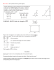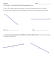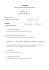# Algebra with shapes How to . . .

## Transcription

Algebra with shapes How to . . .
```Algebra with shapes
Now have a go yourself . . .
(1) The diagram shows a triangle inside a rectangle. All
How to . . .
measurements are given in centimetres.
ABCD is a square with a
side length of 4x.
M is the midpoint of DC.
N is the point on AD where
ND = x
BMN is a right-angled triangle.
Find
an
expression,
in
terms of x, for the area of triangle BMN. Give your expression in its simplest form.
Show that the total area, in cm2, of the shaded regions is
18x - 30
(2) The diagram shows a pentagon. All measurements are
given in centimetres.
(4)
Show that the area of this pentagon can be written as
5x2+x - 6
www.justmaths.co.uk
Exam Questions
Checklist
Working out shown
Keywords
ABCD is a rectangle.
X is the midpoint of AB.
Y is the midpoint of BC.
Z is the midpoint of CD.
What fraction of the total area of ABCD is shaded?
Things to remember ...
What went well ...
(4)
Teacher comment ..
```

### Solve the problems below to answer the riddle: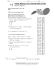### The Midpoint Formula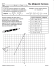### Coordinate Geometry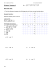### 5.1 Midsegment Theorem and Coordinate Proof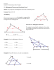### Time: 3 to 3 ½ hours M.M.:90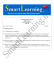### ABCD Coordinator – Race To The Top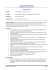### 1-2 Practice A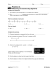### Lesson 30: Special Lines in Triangles M1 Lesson 30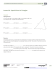### Guidelines TV Lab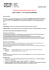### Geometry Sample Questions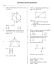### MATHEMATICS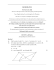### Sample Question Paper Term - II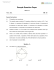### Geometry Chapter 6 REVIEW Problems 3/4/2015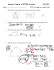### Algebra: Simplifying Algebraic Expressions, Expanding Brackets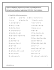### HSM12CC_GM_06_09_CM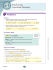### Reteach Sec 1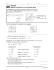### Document 6526483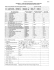### Document 6532627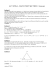### MINISTERUL EDUCAŢIEI AL REPUBLICII MOLDOVA Îţi dorim mult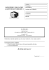### MA.7.A.1.3: Scale Factor